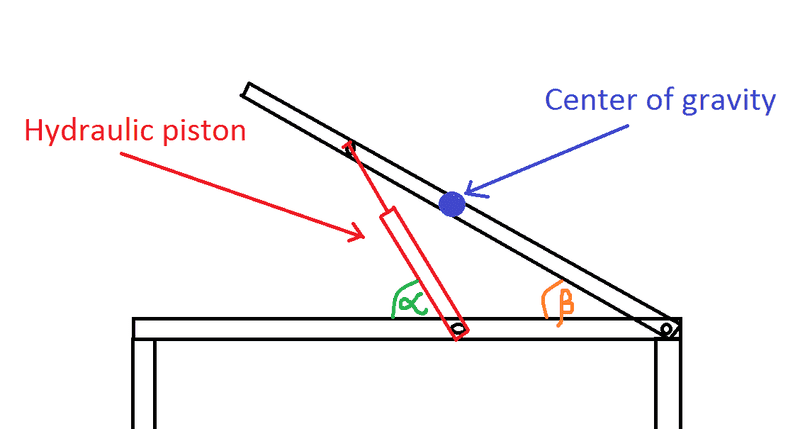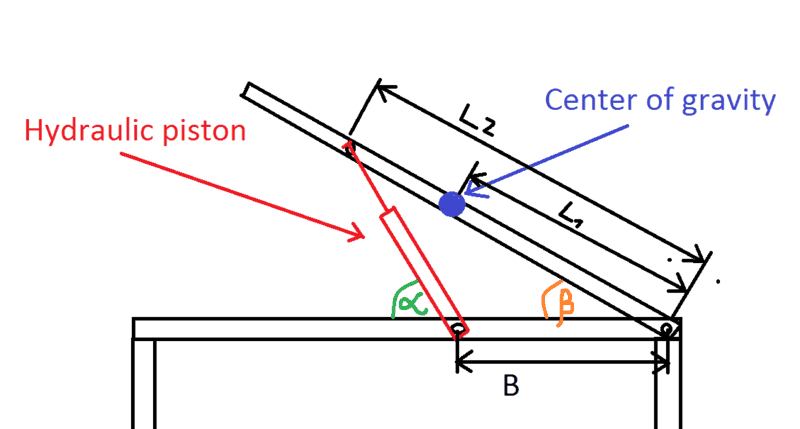# Hydraulic piston force as a function of angle for a launcher

Hello,

A friend of mine challenged me with a question related to erection of missile launcher. For some reason and after too long struggling with it i gave up and trying now to figure out what is the point that i'm missing. The task is to fine the force that the piston is "feeling" as function of the angle of the launcher. I have attached a picture. Can someone provide me an elaborated solution?

In addition, i would like to have a graph of this relation between force and angle?
I got lost with the trigonometry...

Best regards,

KerilYou need to specify the dimensions (distance between pivots, etc) before this can be attacked. With that information given, then there is a bit of kinematics required and the application of the principle of Virtual Work will lead directly to the desired result.

I see. So i have added parameters. how can virtual work be done?To apply the principal of Virtual Work, you need to begin with a generalized coordinate (I'd use the length of the hydraulic system). Then calculate the virtual work done due to a change in the generalized coordinate. In order to do this, you will first need to relate the height of the CM to the generalized coordinate, a kinematics problem.

where can i find a similar problem with a virtual work solution?

There is a somewhat similar problem solved by virtual work in Mechanics of Machines by Doughty (Wiley, 1988), p. 223. This is part of a chapter on Statics by Virtual Work.

thank you for that information. unfortunately. i do not have an access to the library anymore. can you try to solve this problem parametriclly? I find this problem kind of complicated because the force direction is changing all the time and the beam angle is changing as well... i'm trying to use virtual work, and need to take the virtual motion multiplied by the displacement on the same direction....it is changing all the timeThe key to this problem, and so many others, is int the kinematics. Let's talk about that first.

Let q denote the length of the hydraulic unit. Then the following equations are true from the triangle:
B + q*cos(alpha) = L2*cos(beta)
q*sin(alpha) = L2*sin(beta)
Can you solve this system to express beta = f(q)?

Assuming that you can then you can write
Ycm = L1*sin(beta)
V = W*Ycm - potential energy
F * delta q - delta V = 0 - virtual work done on the system
Form the necessary variations and solve for F, the piston force.

•Avichal Tiwari